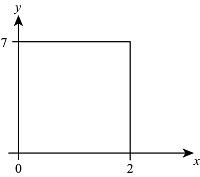# Suppose the temperature in degrees Celsius on the surface of a metal plate is f(X, Y) = 60 -...

## Question:

Suppose the temperature in degrees Celsius on the surface of a metal plate is {eq}f(X, Y) = 60 - 4x^2 - y^2, {/eq} where times and y are measured in centimeters. Estimate the average temperature if times varies between 0 and 2 centimeters and y varies between 0 and 7 centimeters.

## Temperature:

It helps us to find how much any object is hot or cold. It is an absolute property that arises due to intermolecular collision. The difference of this property leads to the transfer of energy. It is denoted by SI unit Kelvin(K).

Given data:• Equation of given function is, {eq}f\left( {x,y} \right) = 60 - 4{x^2} - {y^2} {/eq}
• {eq}\begin{align*} &0 \le x \le 2\\ &0 \le y...

Become a Study.com member to unlock this answer! Create your account